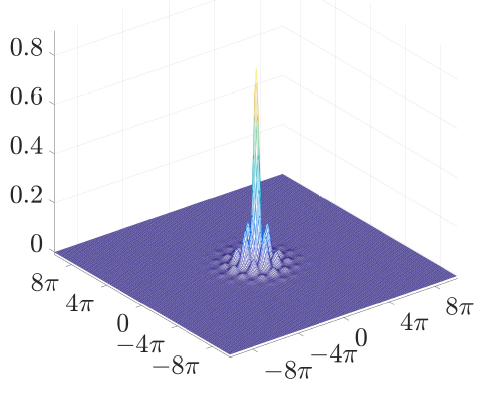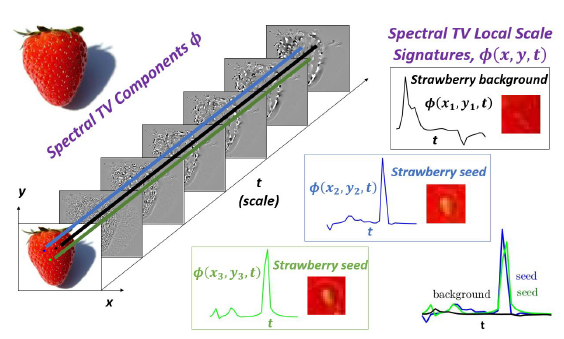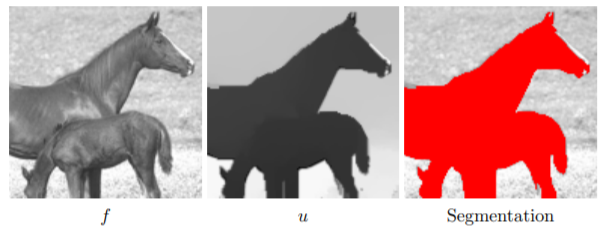# Energy Dissipating Flows for Solving Nonlinear Eigenpair Problems

### Ido Cohen, Guy Gilboa, “Energy dissipating flows for solving nonlinear eigenpair problems”, Journal of Computational Physics 375 (2018), 1138-1158.

This work is concerned with computing nonlinear eigenpairs, which model solitary waves and various other physical phenomena. We aim at solving nonlinear eigenvalue problems of the general form
$T(u)=\lambda Q(u)$. In our setting $T$ is
a variational derivative of a convex functional (such as the Laplacian operator with respect to the Dirichlet energy),
$Q$ is an arbitrary bounded nonlinear operator and $\lambda$ is an unknown (real) eigenvalue.
We introduce a flow that numerically generates an eigenpair solution by its steady state.

Analysis for the general case is performed, showing a monotone decrease in the convex functional throughout the flow.
When $T$ is the Laplacian operator, a complete discretized version is presented and analyzed. We implement our algorithm on \ac{KdV} and \ac{NLS} equations in one and two dimensions.
The proposed approach is very general and can be applied to a large variety of models. Moreover, it is highly robust to noise and to perturbations in the initial conditions, compared to classical Petiashvili-based methods.# Spectral Total-Variation Local Scale Signatures for Image Manipulation and Fusion

### Ester Hait, Guy Gilboa, “Spectral Total-Variation Local Scale Signatures for Image Manipulation and Fusion”, preprint, HAL 01722459, 2018.

We propose a unified framework for isolating, comparing and differentiating objects within an image. We rely on the recently proposed total-variation transform, yielding a continuous, multi-scale, fully edge-preserving, local descriptor, referred to as spectral total-variation local scale signatures. We show and analyze several useful merits of this framework. Signatures are sensitive to size, local contrast and composition of structures; are invariant to translation, rotation, flip and linear illumination changes; and texture signatures are robust to the underlying structures. We prove exact conditions in the 1D case. We propose several applications for this framework: saliency map extraction for fusion of thermal and optical images or for medical imaging, clustering of vein-like features and size-based image manipulation.# Theoretical Analysis of Flows Estimating Eigenfunctions of One-homogeneous Functionals

### Jean-Francois Aujol, Guy Gilboa, Nicolas Papadakis, “Theoretical Analysis of Flows Estimating Eigenfunctions of One-homogeneous Functionals”, accepted to SIAM J. on Imaging Sciences.

Nonlinear eigenfunctions, induced by subgradients of one-homogeneous functionals (such as the 1-Laplacian), have shown to be instrumental in segmentation, clustering and image decomposition. We present a class of ows for nding such eigenfunctions, generalizing a method recently suggested by Nossek and Gilboa. We analyze the ows on grids and graphs in the time-continuous and timediscrete settings. For a specic type of ow within this class, we prove convergence of the numerical iterations procedure and prove existence and uniqueness of the time-continuous case. Several toy examples are provided for illustrating the theoretical results, showing how such ows can be used on images and graphs.# A Discrete Theory and Efficient Algorithms for Forward-and-Backward Diffusion Filtering

### Martin Welk, Joachim Weickert, Guy Gilboa, “A Discrete Theory and Efficient Algorithms for Forward-and-Backward Diffusion Filtering”, preprint.

Image enhancement with forward-and-backward (FAB) diffusion lacks a sound theory and is numerically very challenging due to its diffusivities that are negative within a certain gradient range. In our paper we address both problems. First we establish a comprehensive theory for space-discrete and time-continuous FAB diffusion processes. It requires approximating the gradient magnitude with a nonstandard discretisation. Then we show that this theory carries over to the fully discrete case, when an explicit time discretisation with a fairly restrictive step size limit is applied.
To come up with more efficient algorithms we propose three
accelerated schemes:
(i) an explicit scheme with global time step size adaptation that is
also well-suited for parallel implementations on GPUs,
(ii) a randomised two-pixel scheme that offers optimal adaptivity of
the time step size,
(iii) a deterministic two-pixel scheme which benefits from less restrictive
consistency bounds.
Our experiments demonstrate that these algorithms allow speed-ups by up to three orders of magnitude without compromising stability or introducing visual artifacts.